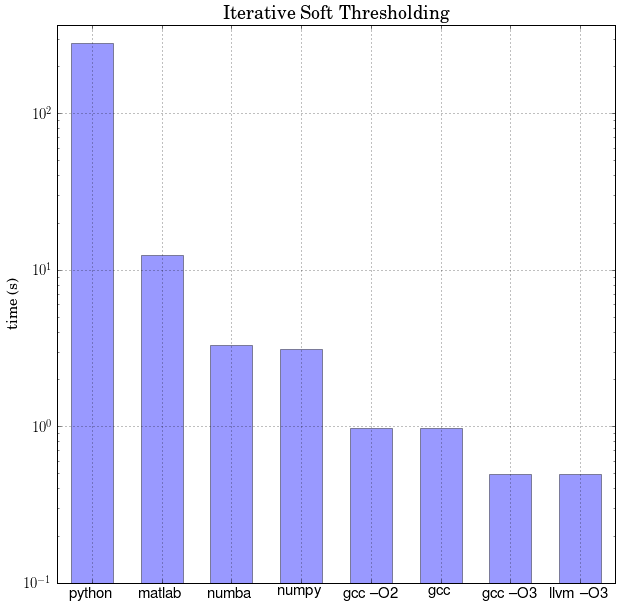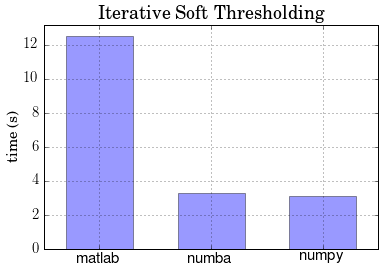# Python, NumPy, Numba, Matlab and C¶

In my field, signal processing, Matlab is heavily used, and I see Python as becoming a valid competitor, eventually. However, one of the advantages of Matlab is that it has a just-in-time compiler (JIT), making it much faster. Here, I test to see how much faster. I use Python's JIT, Numba, as well, and also include a C file to compare against.

Any file compared is viewable at my GitHub. It's over 800 lines of code, the reason it's not in this notebook. As for overhead, I've tested the notebook GUI out, and there's no added overhead.

This notebook is best viewed at nbviewer.ipython.org.

My field is called compressed sensing. It's where you reconstruct missing data from existing structure. For example, you know that your signal doesn't have an infinite number of changes. You use this fact to your advantage, minimizing the number of changes an in image. This relies on the fact that many areas in a picture are nearly solidly colored. The algorithm, iterative soft thresholding (IST), is complex, so I'll just show you an example of what it can do.

In :
from IST import *

sample = zeros_like(I)
for i in arange(3):
x, ys = ISTreal(I[:,:,i], its=100, p=0.5)
# takes around 20 minutes on this machine
I[:,:,i] = idwt2_full(x)
sample[:,:,i] = ys

figure(figsize=(14,14))
subplot(121)
imshow(sample)
axis('off')
title('The sampled image')

subplot(122)
imshow(I)
axis('off')
title('The reconstructed image')

show()As you can see, a fairly good reconstruction. This algorithm is widely used in my field, and is an iterative process, good for testing JITs. So now, we'll get onto the meat of this notebook and test it the speed of this in Python, NumPy, Numba, Matlab and C.

### Python¶

Here, we're going to define a several functions (discrete wavelet operations, inverse and forward), then test the iterative soft thresholding (IST). You can see all the declarations in the GitHub repo.

This pure python module uses for-loops as well as Numpy, but Numpy is famously bad at for-loops.

In :
%%capture string
import ISTpython as ISTp
#%timeit ISTp.IST()
# it takes a long time for this to finish

In :
#print string.stdout
#python = float(string.stdout.split()[-4])
string = '1 loops, best of 3: 283 s per loop'
print string
python = float(string.split()[-4])

1 loops, best of 3: 283 s per loop


### NumPy¶

The only change we make is that we vectorize our for-loops in our two fundamental functions (done thanks to a Reddit comment. Instead of

for i in arange(16):
y[i]   = x[2*i] + x[2*i+1]
y[i+l] = x[2*i] - x[2*i+1]



we have

i = arange(16)
y[i]   = x[2*i] + x[2*i+1]
y[i+l] = x[2*i] - x[2*i+1]



I also vectorized dwt2 and idwt2, a more difficult task. I had to use zeros_like, not zeros(len(x)).

In :
%%capture string
import IST as ISTnumpy
%timeit ISTnumpy.IST()

In :
print string.stdout
numpy = float(string.stdout.split()[-4])

1 loops, best of 3: 3.1 s per loop



### Numba Wrapper¶

If you have good NumPy code, Numba is easy to integrate. If you do anything unusual (play with strings, tuples, keyword args), you get errors but those will (hopefully) be fixed soon.

The functions in ISTnumba the functions in IST with an @autojit decerator.

In :
%%capture string
import ISTnumba
%timeit ISTnumba.IST()

In :
print string.stdout
numba = float(string.stdout.split()[-4])

1 loops, best of 3: 3.28 s per loop



### MATLAB¶

Here, I'm using Matlab's tic and toc functions to time it.

This Matlab code can be optimized more. I tried using parfor to excute my loops in dwt2 and idwt2 in parallel. I couldn't load the proper toolbox and get the proper liscense.

In :
string = !matlab -nodisplay -nosplash -r "run IST.m; exit"
string = string[-1]
print string

matlab = float(string.split()[-2])

Elapsed time is 12.505537 seconds.


### C¶

And the C versions, with the optimization flags and various compilers (all from the same C file, of course).

In :
!gcc IST.c -o IST.o
string = !time ./IST.o
c = float(string.split()[2:-1])
print "Total time in seconds: ", c

Total time in seconds:  0.976

In :
!gcc -O2 IST.c -o IST_O2.o
string = !time ./IST.o
c_O2 = float(string.split()[2:-1])
print "Total time in seconds: ", c_O2

Total time in seconds:  0.979

In :
!gcc -O3 IST.c -o IST_O3.o
string = !time ./IST_O3.o
c_O3 = float(string.split()[2:-1])
print "Total time in seconds: ", c_O3

Total time in seconds:  0.513

In :
!llvm-gcc -O3 IST.c -o IST_llvm.o
string = !time ./IST_llvm.o
c_llvm = float(string.split()[2:-1])
print "Total time in seconds: ", c_llvm

Total time in seconds:  0.495

In :
!mpicc -O3 ISt.c -o ISTparallel.o
string = !time ./ISTparallel.o
mpicc = float(string.split()[2:-1])
print "Total time in seconds: ", mpicc

Total time in seconds:  0.494


### Graphing¶

Of course, we have plots.

In :
from pylab import *

labels = ['python',  'numpy', 'numba', \
'matlab', 'gcc', 'gcc --O2', \
'gcc --O3', 'llvm --O3']
timings = [python, numpy, numba, matlab, c, c_O2, c_O3, c_llvm]
x = np.arange(len(labels))

# plotting from slowest to fastest
i = argsort(timings)
timings = sort(timings)[::-1]
labels = asarray(labels)
labels = labels[i][::-1]
fastest = timings[-1]

figure(figsize=(10,10))
matplotlib.rcParams.update({'font.size': 15})
ax = plt.axes(xticks=x, yscale='log')
ax.bar(x - 0.3, timings, width=0.6, alpha=0.4, bottom=1E-6)
ax.grid()
ax.set_xlim(-0.5, len(labels) - 0.5)
ax.set_ylim(1E-1, python*1.3)
ax.xaxis.set_major_formatter(plt.FuncFormatter(lambda i, loc: labels[int(i)]))
ax.set_ylabel('\\textrm{time (s)}')
ax.set_title("\\textrm{Iterative Soft Thresholding}")
show()And zooming closer in on the range we're interested in, and doing those comparisions... (with a linear, not log, scale!)

In :
labels  = ['numpy', 'numba', 'matlab']
timings = [ numpy,   numba,   matlab]
x = np.arange(len(labels))

# plotting from max to min times
i = argsort(timings)
timings = sort(timings)[::-1]
labels = asarray(labels)
labels = labels[i][::-1]

ax = plt.axes(xticks=x)#, yscale='log')
ax.bar(x - 0.3, timings, width=0.6, alpha=0.4, bottom=1E-6)
ax.grid()
ax.set_xlim(-0.5, len(labels) - 0.5)
ax.set_ylim(0, 1.05*max(timings))
ax.xaxis.set_major_formatter(plt.FuncFormatter(lambda i, loc: labels[int(i)]))
ax.set_ylabel('\\textrm{time (s)}')
ax.set_title("\\textrm{Iterative Soft Thresholding}")
show()In :
print "C comparisions:"
print "NumPy / C  :", numpy  / fastest
print "Numba / C  :", numba  / fastest
print "Matlab / C :", matlab / fastest

print "\nThe comparision that we care about, NumPy and Matlab:"
print "Matlab / NumPy: ", matlab / numpy
print "Matlab / Numba: " , matlab / numba

C comparisions:
NumPy / C  : 6.26262626263
Numba / C  : 6.62626262626
Matlab / C : 25.2637111111

The comparision that we care about, NumPy and Matlab:
Matlab / NumPy:  4.03404419355
Matlab / Numba:  3.81266371951


It should be noted that Matlab has had a JIT compiler since 2002, and Numba is a recent development that was only started a year ago (in Mar. 2012). My sense is that it will go the way of IDL, another high-level and technical programming language that cost thousands, and is all but extinct now. The freedom for anyone to run your code and encourages collaboration, the reason I don't think Python will go the way of Matlab.

As it stands, autojit doesn't add much if anything when the code depends on low level libraries.

### My experience with Python¶

Because your code is only fast if it's optimized, it's hard to program. For example, while prototyping code, I had to depend on a for loop in Python -- something really slow, meaning it took me about 30 minutes to get feedback/results. The same code in Matlab would have been much, much faster since this test is essentially the same code as plain Python. For prototyping, I say use Matlab.

Will I still write my final programs in Python? Yes. Will I still write sk-learn functions? Yes. Do I like using Matlab? No.

In :